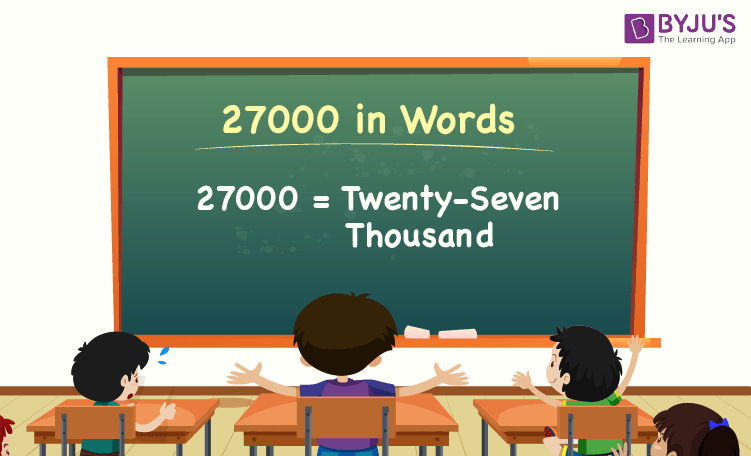27000 in words is Twenty-seven Thousand. The number name of 27000 is written using the place value of a number, such as ones, tens, hundreds, thousands, etc. For example, if you have saved 27000 in a month, you can write, “I have saved twenty-seven thousand in a month”.

 27000 in Words: Twenty-seven Thousand

In this article, we will learn how to write 27000 in words, i.e., the spelling of 27000 in words, and look at many solved examples.

## How to Write 27000 in Words?The place value chart helps to write the number 27000 in words. Using the ones, tens, hundreds, and thousands place value of each digit in the number 27000, we can easily write the number name of 27000.

For a number 27000,

The digit in one’s place = 0

The digit in ten’s place = 0

The digit in hundred’s place = 0

The digit in thousand’s place = 27

Thus, 27000 is represented in the place value chart as follows:

 Thousands Hundreds Tens Ones 27 0 0 0

Therefore, the number 27000 in words is Twenty-seven Thousand.

### Examples

Example 1:

Determine the value of 24000 + 3000. Express the value in words.

Solution:

Given expression: 24000 + 3000

⇒ 24000 + 3000 = 27000

So, the value of 24000 + 3000 is 27000.

Hence, 27000 in words is twenty-seven thousand.

Example 2:

What is the value of twenty-seven thousand plus two thousand in words?

Solution:

Twenty-seven thousand = 27000

Two thousand = 2000

Twenty-seven thousand plus two thousand = 27000 + 2000 = 29000

Hence, the value of twenty-seven thousand plus two thousand is twenty-nine thousand in words.

## Frequently Asked Questions on 27000 in Words

Q1

### Write 27000 in words.

27000 in words is twenty-seven thousand.

Q2

### What is the value of 27500 – 500? Express the value in words.

Simplifying 27500 – 500, we get 27000. Hence, 27000 in words is twenty-seven thousand.

Q3

### How to write twenty-seven thousand in numbers?

Twenty-seven thousand in numbers is 27000.

Q4

### Is 27000 an even number?

Yes, 27000 is an even number.

Q5

### Is 27000 a prime number?

No, 27000 is not a prime number.